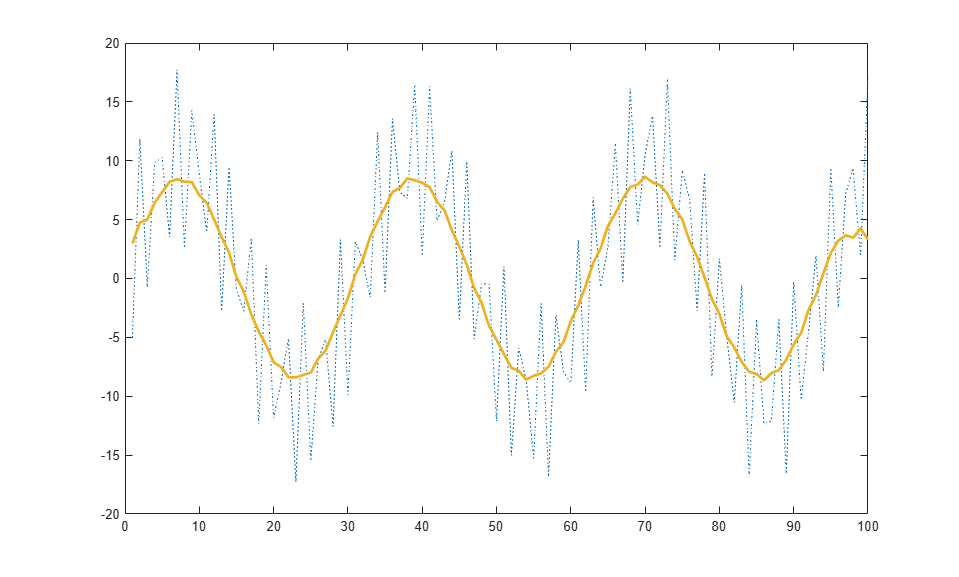# setup

Class: matlab.graphics.chartcontainer.ChartContainer
Package: matlab.graphics.chartcontainer

Set up instance of chart container subclass

## Syntax

```setup(obj) ```

## Description

`setup(obj)` sets the initial state of the chart. It executes once when the chart object is created. Any property values passed as name-value pair arguments to the chart's constructor method are assigned after the `setup` method executes.

Define this method to execute initialization code for each new instance of your class. For example, you can use this method to create the underlying graphics objects and set initial property values on those objects.

## Input Arguments

expand all

Object of the class that inherits from the `matlab.graphics.chartcontainer.ChartContainer` base class.

## Attributes

 `Abstract` `true` `Protected` `true`

To learn about attributes of methods, see Method Attributes.

## Examples

expand all

Define a class called `SmoothPlot` that plots a set of data using a dotted blue line with a smoothed version of the line.

To define the class, create a file called `SmoothPlot.m` that contains the following class definition with these features:

• `XData` and `YData` public properties that store the x- and y-coordinate data for the original line.

• `SmoothColor` and `SmoothWidth` public properties that control the color and width of the smooth line.

• `OriginalLine` and `SmoothLine` private properties that store the `Line` objects for original and smoothed data.

• A `setup` method that initializes `OriginalLine` and `SmoothLine`.

• An `update` method that updates the plot when the user changes the value of a property.

• A `createSmoothData` method that calculates a smoothed version of `YData`.

```classdef SmoothPlot < matlab.graphics.chartcontainer.ChartContainer properties XData (1,:) double = NaN YData (1,:) double = NaN SmoothColor (1,3) double {mustBeGreaterThanOrEqual(SmoothColor,0),... mustBeLessThanOrEqual(SmoothColor,1)} = [0.9290 0.6940 0.1250] SmoothWidth (1,1) double = 2 end properties(Access = private,Transient,NonCopyable) OriginalLine (1,1) matlab.graphics.chart.primitive.Line SmoothLine (1,1) matlab.graphics.chart.primitive.Line end methods(Access = protected) function setup(obj) % Create the axes ax = getAxes(obj); % Create the original and smooth lines obj.OriginalLine = plot(ax,NaN,NaN,'LineStyle',':'); hold(ax,'on') obj.SmoothLine = plot(ax,NaN,NaN); hold(ax,'off') end function update(obj) % Update line data obj.OriginalLine.XData = obj.XData; obj.OriginalLine.YData = obj.YData; obj.SmoothLine.XData = obj.XData; obj.SmoothLine.YData = createSmoothData(obj); % Update line color and width obj.SmoothLine.Color = obj.SmoothColor; obj.SmoothLine.LineWidth = obj.SmoothWidth; end function sm = createSmoothData(obj) % Calculate smoothed data v = ones(1,10)*0.1; sm = conv(obj.YData,v,'same'); end end end ```

Next, create a pair of `x` and `y` vectors. Plot `x` and `y` by calling the `SmoothPlot` constructor method, which is provided by the `ChartContainer` class. Specify the `'XData'` and `'YData'` name-value pair arguments and return the object as `c`.

```x = 1:1:100; y = 10*sin(x./5) + 8*sin(10.*x + 0.5); c = SmoothPlot('XData',x,'YData',y); ```Use `c` to change the color of the smooth line to red.

```c.SmoothColor = [1 0 0]; ```## Compatibility Considerations

expand all

Behavior changed in R2020a